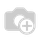# How to pass field data one model to another model.

How to pass field data one model to another model.

class one(model.Model):

_name = one.one

x = fields.Integer()

y = fields.Integer()

class two(model.Model):

_name = two.two

p = fields.Integer()

k = fields.Integer()

here i need x field valueHello Arjun,

If you want to get data from model one.one's field in model two.two's field, then just take many2one of one.one model in two.two and take related filed of it, let me explain by example:

class one(models.Model):

_name = one.one

x = fields.Integer()

y = fields.Integer()

class two(models.Model):

_name = two.two

one_id = fields.Many2one('one.one', string='One')

x = fields.Integer(related='one_id.x', string="X")

y = fields.Integer()

Regards,Email:      odoo@aktivsoftware.com

Skype: kalpeshmaheshwari

1 Commentx field is computed field that data is stored in database now i want to pass x field data in the second model. but your above method is not working